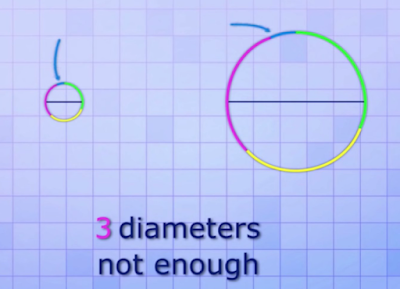# Where did the circle come from?

Where did the circle come from? – We all know = 3.14… or 22/7 However, few of us know how that number can be obtained. Our “basically memorized” school system is pretty bad. In fact, explaining it is quite easy and makes us understand the logic behind it. Not all teachers can or want to teach things like this. Many creative and intelligent children do not want to be teachers. At the end, many who become teachers are mediocre. Having a teacher who is creative, smart and has good communication is a privilege.

I admit that education now only wants to be simple and what is important without knowing the process. I still remember always asking how did you get this? The answer was that I just followed the time, I just didn’t know and in the end I was labeled a fool

But to be honest, after I lived a long time and went through a series of questions that I kept to myself until I found the answers, I immediately felt “this is how it is in books”

But when I found the answer I wanted, I was very satisfied

Where did the Phi Circle come from?

*simple:

To understand , please look at the image below.

is obtained by dividing the circumference by the diameter of the circle itself. No matter how big/small the circle is, the ratio remains the same: 3.14…

To prove it, you can make a loop of thread / cable freely. Then, take one more thread to measure the diameter.

If so, try to take the thread to measure the diameter and calculate the circumference of the circle. The steps are as shown below.It’s that simple, there are many formulas and other mathematical notations that are easy to understand, but we usually just memorize them right away.

Now, it will be easier for us to imagine formulas that use , for example:

Circle area: r^2

Cube volume: 4/3 r^3

Or even the formulas we see in probability and statistics here.

Another way:

In the past, at Mts, they were taught to look for “π” (pi/phi) using the algebraic system

So here’s what it’s like (sorry if it’s wrong and complicated haha):

– Make a circle from cardboard (try to be symmetrical) with a random diameter (free)

– Take 1 strand of thread and then circle the circle that was made earlier

– Now just calculate how long the diameter is by folding the circle earlier, fold it 2 symmetrically

– Here we already have 2 numbers, namely the diameter and the circumference of the circle, then we can start solving the problem

But we know that with manual calculations, the circumference and diameter are not always in the right numbers (special numbers) so the results are sometimes not as accurate as the existing formula, more or less 80%-99% similar to the existing formula.

For example, let’s take a special number

Perimeter (C) = 44 CM

Diameter (d) = 14 CM

We have to find out why with a diameter of 14 CM the circumference is 44 CM, because the diameter number is smaller than the circumference, we have to increase the value of the diameter by adding or multiplying it.

Now we describe with algebra, why algebra? because it is adapted to its function to solve mathematical problems.

Let’s try one sentence:

How many numbers are needed (n) when multiplied by the diameter (d) of the circle, the result is the circumference (C) of the circle itself?

We describe:

n × d = C

Means

n = C d

We lower the numbers:

n × 14 CM = 44 CM

Means

n = 44 CM 14 CM

There we know that the number needed for the diameter multiplier is called “n”, now “n” is symbolized by “π” (pi/phi)

and believe me any number 1 or number 2 as a real number is only limited to “approaching” we do not know for sure what is 1 or what is 2 which essentially forms it but if you use anti-realist theory 1 is “ADA” because “ADA” is not 1, the concept of one and multiple from alain badiou

A fun fact about is that can be written to an infinite number of times. Any combination may be there, your date of birth, when you will meet your soulmate, until the day you die can be written there.

.

Published
Categorized as Edukasi